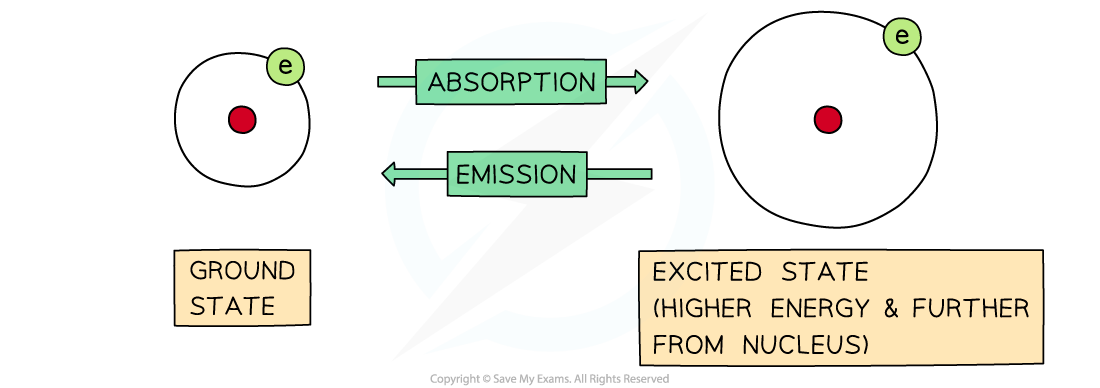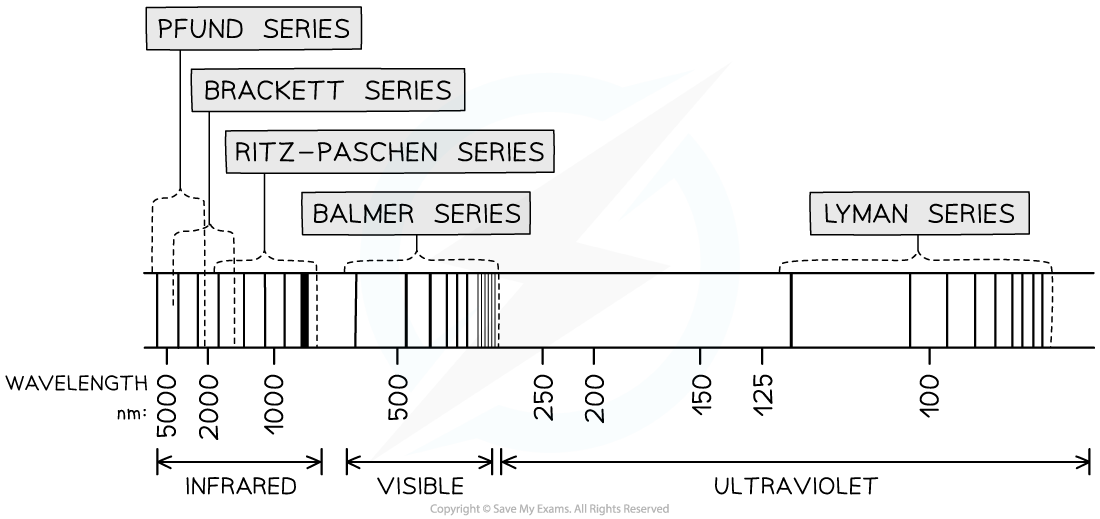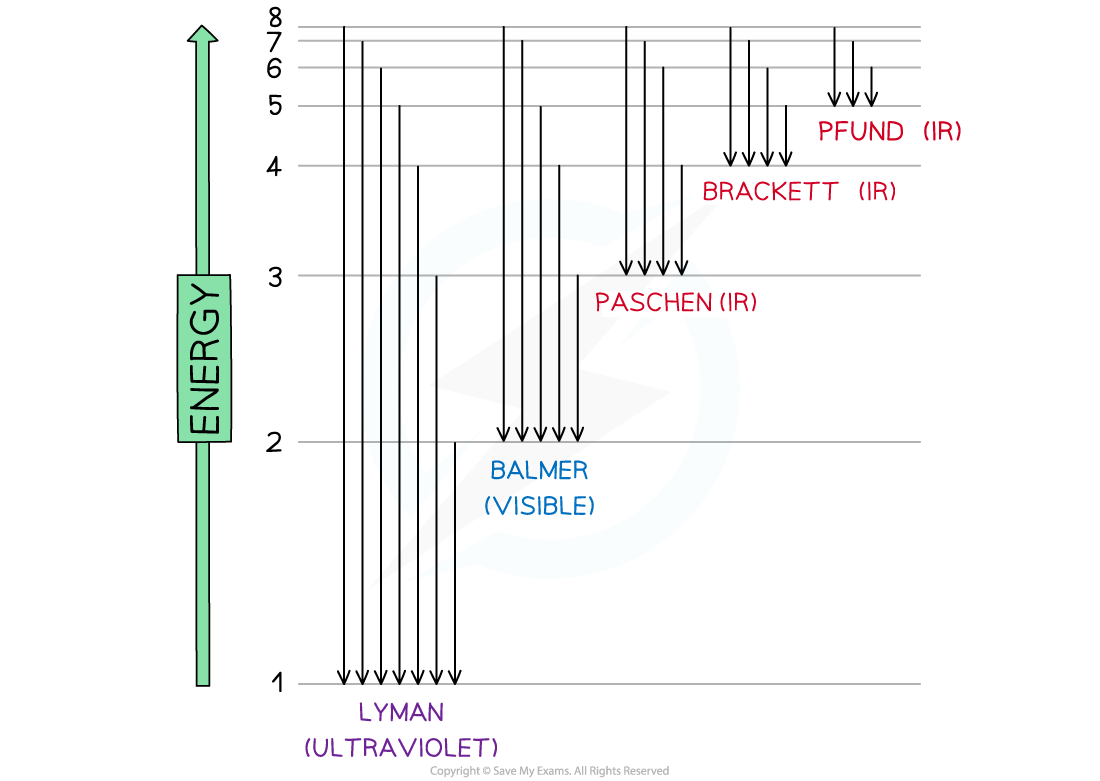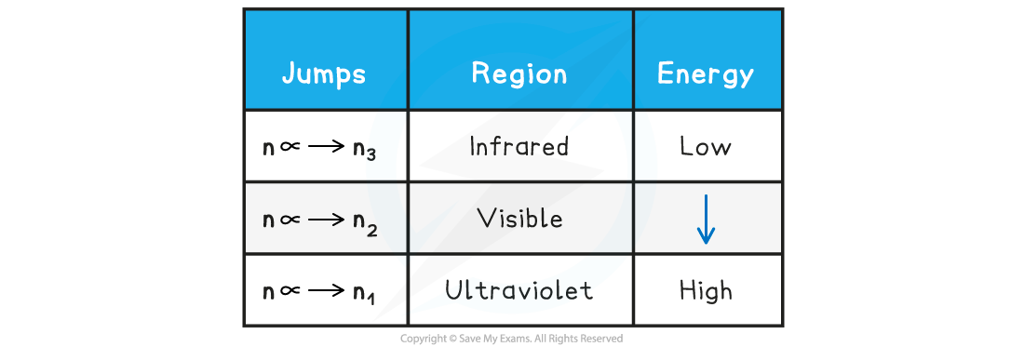# IB DP Chemistry: SL复习笔记2.1.5 Emission Spectra

### Emission Spectra

• Electrons move rapidly around the nucleus in energy shells
• If their energy is increased, then they can jump to a higher energy level
• The process is reversible, so electrons can return to their original energy levels
• When this happens, they emit energy
• The frequency of energy is exactly the same, it is just being emitted rather than absorbed:The difference between absorption and emission depends on whether electrons are jumping from lower to higher energy levels or the other way around

• The energy they emit is a mixture of different frequencies
• This is thought to correspond to the many possibilities of electron jumps between energy shells
• If the emitted energy is in the visible region, it can be analysed by passing it through a diffraction grating
• The result is a line emission spectrum

#### Line emission spectraThe line emission (visible) spectrum of hydrogen

• Each line is a specific energy value
• This suggests that electrons can only possess a limited choice of allowed energies
• These packets of energy are called 'quanta' (plural quantum)
• What you should notice about this spectrum is that the lines get closer together towards the blue end of the spectrum
• This is called convergence and the set of lines is converging towards the higher energy end, so the electron is reaching a maximum amount of energy
• This maximum corresponds to the ionisation energy of the electron
• These lines were first observed by the Swiss school teacher Johannes Balmer, and they are named after him
• We now know that these lines correspond to the electron jumping from higher levels down to the second or n = 2 energy level

### The Hydrogen Spectrum

• A larger version of the hydrogen spectrum from the infrared to the ultraviolet region looks like thisThe full hydrogen spectrum

• In the spectrum, we can see sets or families of lines
• Balmer could not explain why the lines were formed - an explanation had to wait until the arrival of Planck's Quantum Theory in 1900
• Niels Bohr applied the Quantum Theory to electrons in 1913, and proposed that electrons could only exist in fixed energy levels
• The line emission spectrum of hydrogen provided evidence of these energy levels and it was deduced that the families of lines corresponded to electrons jumping from higher levels to lower levelsElectron jumps in the hydrogen spectrum

• The findings helped scientists to understand how electrons work and provided the backbone to our knowledge of energy levels, sublevels and orbitals
• The jumps can be summarised as follows:

Electron Jumps & Energy Table#### Worked Example

Which electron transition in the hydrogen atom emits visible light?

A. n = 1 to n = 2

B. n = 2 to n = 3

C. n = 2 to n = 1

D. n = 3 to n = 2

Option D is correct

• Emission in the visible region occurs for an electron jumping from any higher energy level to n = 2